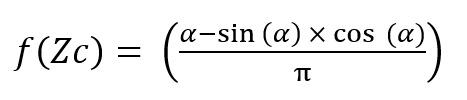# How to Calculate Partial Volume of Horizontal Vessel with Ellipsoidal Heads

In this post, I want to share with you how to calculate partial volume of horizontal vessel with ellipsoidal heads. Basically, the horizontal vessel consists of cylinder and two heads. Thus, the total volume will be:

Total volume = volume of liquid in two heads + volume of liquid in cylinder
Total volume = 1/6 π K1 D3 + 1/4 π D2 L

Where:

K1 = 2 b/D

b, D, and L are function of vessel geometry. Please see figure below.

On the other hand, partial volume is calculated using equation below:

Partial volume = 1/6 π K1 D3 × [f(Ze)] + 1/4 π D2 L × [f(Zc)]

Where:

f(Ze) = ellipsoidal coefficient            orf(Zc) = horizontal cylinder coefficient     orFor ellipsoidal head, b = ¼ D, K1 = ½